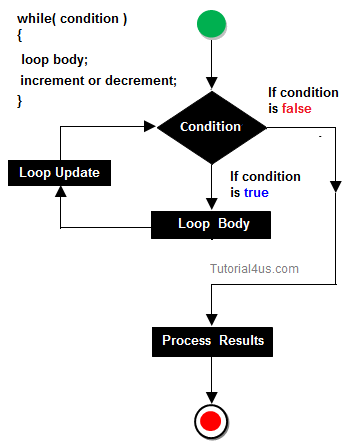### While loop:

The most basic loop in JavaScript is the while loop. There are two key parts to a JavaScript while loop:

The conditional statement which must be true for the while loop's code to be executed.
The while loop's code that is contained in curly braces"{and}" will be executed if the condition is true.

When a while loop begins, the JavaScript interpreter checks if the condition statement is true. If it is, then the code between the curly braces is executed.

The same procedure is repeated until the condition stays true. If the condition statement is always True, then you will never exit the while loop.

### Syntax:

```
while(expression)
{
Statement(s) to be executed if expression is tru
}

```

### Flow Chart:```
sum=1275

```

### Result :

```  loop = 0
loop = 1
loop = 2
loop = 3
loop = 4

```